Journal of Energy and Natural Resources
Volume 5, Issue 1-1, February 2016, Pages: 1-5

A Variable Step Size MPPT Method for Stand-Alone PV Energy Systems

Tahar Tafticht, Yamina Azzoug

Electrical Engineering Department, College of Engineering, Majmaah University, Majmaah, KSA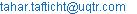(T. Tafticht)

Tahar Tafticht,Yamina Azzoug. A Variable Step Size MPPT Method for Stand-Alone PV Energy Systems. Journal of Energy and Natural Resources. Special Issue: Electrical Power Resources: Coal versus Renewable Energy. Vol. 5, No. 1-1, 2016, pp. 1-5. doi: 10.11648/j.jenr.s.2016050101.11

Abstract: This paper presents an optimal algorithm control of photovoltaic generator system with battery energy storage. The system is the combination of photovoltaic (PV) array and battery storage via a common dc bus. The system components have substantially different voltage-current characteristics and they are integrated on the DC bus through power conditioning devices for optimal operation by using the developed Maximum Power Point Tracking (MPPT) control method. Using this method, it is possible to adapt the load to the PV modules and to follow the MPP howsoever the weather conditions may vary. This algorithm is based on perturbation and Observation (P&O) method with initial measurement of the tracking reference for estimating the step size to get the optimal operating point. The results show that the approach improves clearly the tracking efficiency of the maximum power available at the output of the PV modules and reduces the oscillations around the maximum power point.

Keywords: Photovoltaic, Battery Energy Storage, MPPT Method

Contents

1. Introduction

PV energy is a clean energy which has had a fast development for the last two decades. However, the intermittent character of the solar irradiation requires the use of some energy storage device so as to be able to continuously supply loads from the PV generators. PV energy seems to be an interesting alternative that makes possible to control the load demand energy and to produce not polluting fuel usable in transportation or the buildings, and thus to diversify the energy markets. There are some previous works on PV energy systems [1-11]. Several publications tackle the problem concerning the optimal design and the sizing of the PV systems [1-5]. The majority of these publications show that the PV power system therefore has higher availability to deliver continuous power and results in a better utilization of power conversion and control equipment than either of the individual sources. In addition, the cost of PV systems, based on long terms of data hourly, daily, and yearly was analyzed [6-11]. The price of PV energy remains high and the energetic efficiency of the installations is relatively low. In order to be useable in a broad reach of applications, and to satisfy the economic constraints, the conversion chain of this energy must be robust and reliable. It must also present a better efficiency and be realized at low cost. For that, it is necessary to extract the maximum power from renewable sources. The operating power of renewable sources depends on the sun intensity, the temperature and especially output voltage for the PV modules. If the power transfer between renewable sources and the load is not optimal, the total efficiency of PV energy system will be largely affected. The renewable sources can be operated at the maximum power operating point for various conditions by adjusting the PV modules output voltage optimally.

This paper presents a new MPPT method used to achieve the maximum power control of PV system. By acquiring the output power of PV system, the control searches the maximum power point and tracks this point in the event of change of the meteorological conditions, in order to reduce the error between the operating power and the maximum power. The application of the MPPT method to PV systems with battery energy storage shows that it is possible to increase significantly the efficiency of storage because of the improved control of the MPP during changing ambient weather conditions. Using this method, it is possible to adapt the load to the PV modules and to follow the MPP howsoever the weather conditions may vary. This paper presents an optimal algorithm control of photovoltaic generator system with battery energy storage. This algorithm is based on P&O method with initial measurement of the tracking reference for estimating the step size to get the optimal operating point. The new algorithm reduces the oscillations around the maximum power point, and increases the average efficiency of the MPPT obtained.

2. System Configuration

Figure 1 presents the diagram of the different components of the hybrid PV system. The system consists of a 1 kW (peak) solar PV array as primary energy sources. The excess energy with respect to load demand has been stored as batteries storage. The hybrid system components have substantially different voltage-current characteristics and are integrated through the developed power conditioning devices on a 48V DC bus, which allows power to be managed between input power, energy storage and load. The PV generator is controlled by a DC/DC buck converter, where the duty ratio is used as the control means to track the maximum power point. The power generated from the PV generator is supplied to the common DC bus. The different sensors are used to record real time voltages and currents of PV generator, common DC bus, load, and DC-DC converter duty ratio.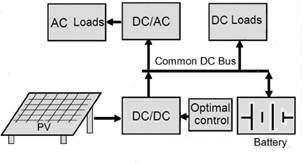Fig. 1. Schematic diagram for the PV system.

3. PV Characteristics

The equivalent electric diagram of a PV array is shown in figure 2. The PV array’s electric characteristic under solar radiation is given by the equation (1) in terms of output current (Ipv) and voltage (Vpv) :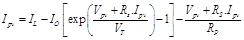(1)

Where IL = Np.Iph corresponds to the light-generated current of the solar array with Iph being the cell light-generated current and Np represents the number of parallel modules.

Io = Np .Ios corresponds to the reverse-saturation current of the solar array, with Ios the cell reverse-saturation current

VT = (Ns.n.KB.T)/q is the thermal voltage, where Ns is the number of cells connected in series, KB is the Boltzmann’s constant, n is the ideality factor, T is the cell temperature, and q is the electron charge;

Rs is the series parasitic resistance of a solar array, Rp its shunt parasitic resistance. The load resistance will be represented by RLoad .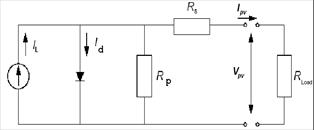Fig. 2. Equivalent electric diagram of a PV array.

An ideal PV module is one for which Rs is zero and Rp is infinitely large. The output current and voltage are then: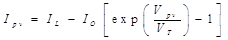(2)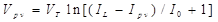(3)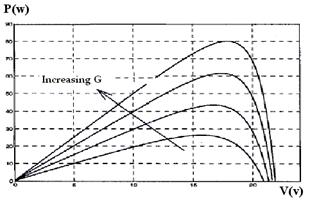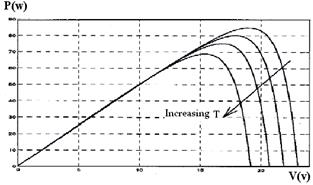b) Temperature influence

Fig. 3. Solar radiation and temperature influences on the P–V characteristics.

The PV array characteristic presents three important points: the short circuit current (Isc), the open voltage (Voc) and the optimum power (Pop) delivered by the PV array to an optimum load (Rop). In this case the PV modules operate at their MPP. Figures 3 a and b give the Power-Voltage (P-V) characteristics of a PV module for varying values of solar radiation and temperature. The short circuit current is clearly strongly proportional to the solar radiation: more radiation, more current, and also more maximum output power. On the other hand the temperature dependence is inverse: an increase in temperature causes a reduction of the open circuit voltage (when sufficiently high) and hence also of the maximum output power. Hence these opposite effects of the variations of solar radiation and temperature on the maximum output power make it important to track the maximum power point efficiently.

4. System Operation

Figure 4 shows the MPPT buck converter diagram for PV optimum power transfer. The switch S of the buck converter is a MOSFET transistor with a low internal resistance Ron. The MOSFET is controlled by a PWM signal generation circuit that uses a microcontroller. For searching the MPP and tracking this point in order to reduce the error between the operating power and the maximum power, in the event of change of the weather conditions, the control of the buck converter track periodically the operating point of the PV module. By acquiring the output voltage and current of PV modules, the control used this information to increase or decrease the duty cycle of the buck converter to change the operating point of the PV array. The operation of the buck converter is described in our previous paper  and Load voltage is given by: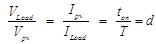(4)

Where ton is turn on period of S switch and T is it period.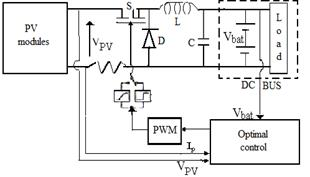Fig. 4. MPPT Buck converter diagram.

5. PV Optimal Control

In order to extract the maximum power, an analysis was provided to understand the probable displacement of the operating point in the two operating zones of PV Generator. Figure 5 represents the typical curve of power variation according to the operating voltage. This figure shows that there are two operating zones: the first is located on the right side of the MPP where dP/dv < 0 and the second on the left side of the MPP where dP/dv > 0.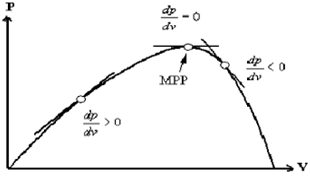Fig. 5. Probable displacement of the operating point.

Figure 6 gives the algorithm of the proposed MPPT control method, where the information on solar radiation or temperature is not required. For searching the maximum power operating point and tracking this point in order to reduce the error between the operating power and the maximum power if the solar radiation or temperature changes, the control of the buck converter perturbs periodically the operating point of the PV Generator. By measuring the output voltage and current of PV Generator, the control uses this information to change the operating point of the PV Generator by increasing or decrease the duty cycle of the buck converter. After the perturbation, there is a displacement of the operating point from (k-1) to (k). Four cases of perturbation from operating point are distinguished:

1st case: If P(k)>P(k-1) and V(k)>V(k-1), the power increases after perturbation. This indicates that the MPP research is oriented to the good direction. So, the search of the MPP continues in the same direction and reaches the operating point (k+1) by increasing the duty cycle by Dd.

2nd case: If P(k)<P(k-1) and V(k)<V(k-1), the power decreases after perturbation. This indicates that the MPP search is oriented to the bad direction. The MPP search direction must be changed and the duty cycle is increased by two Dd to reach the operating point (k+1).

3rd case: If P(k)>P(k-1) and V(k)<V(k-1), the power increases after perturbation. This indicates that the MPP search is oriented to the good direction. Then, the MPP search direction must be maintained and the duty cycle is decreased by one Dd to reach the operating point (k+1).

4rd case: If P(k)<P(k-1) and V(k)>V(k-1), the power decreased. This indicates that the MPP search is oriented to bad direction. The MPP search direction must be changed and the duty cycle is increased by two Dd to reach the operating point (k+1).

To estimate the MPP reference value, the power wand the voltage of the PV modules is measured cyclically at the output solar panels during the opening of the switch of the buck converter. This voltage allows the MPP reference value to be calculated from equation 5, and consequently the value of the converter’s duty cycle (step size) to be adjusted by equation 4. The proposed method scans sequentially the voltage range applicable to the load. At each iteration, the power consumed by the load is calculated. To find the maximum power, the derivative of output power (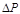) is used. The optimal operating point corresponds to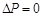.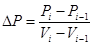(5)

6. Results and Discussion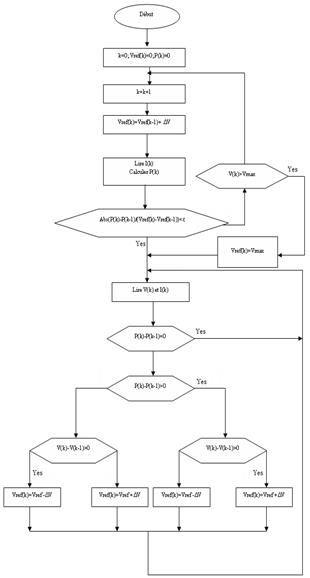Fig. 6. Algorithm of the proposed MPPT control method.

The MPPT control of a PV generator system described above was implemented in a circuit based on a micro-controller PIC18F242 and tested. Figures 7 and 8 give the results obtained with the proposed approach. It is noticed that classic P&O method does not instantly locate the MPP and it has also an oscillation around the MPP (Fig. 7). The new approach reduces the oscillations around the MPP (Fig. 8). This results in almost stable PV voltage and current.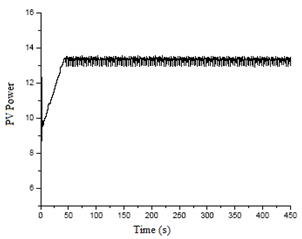Fig. 7. Experimental results of the classic P&O method.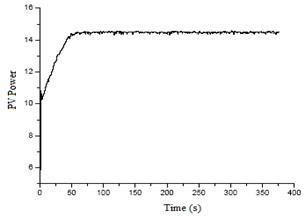Fig. 8. Experimental results of the proposed method.

7. Conclusion

This paper has presented a MPPT Control for efficient operating of PV energy system taking into consideration the operational features of the system. An operational algorithm has been proposed for that purpose, where the information about the temperature and solar radiation is not required. The results show that the approach reduces clearly the oscillations around the maximum power point.

References

1. A. Ashraf, L. Ran and J. Bumby"Simulation and Control of a Hybrid PV-Wind System", Power Electronics, Machines and Drives, 2008. PEMD 2008, York, UK, 2-4 April 2008.
2. F. Valencaga, P. F. Puleston, and P. E. Battaiotto, "Power control of a solar/wind generation system without wind measurement: A passivity/ sliding mode approach," IEEE Trans. Energy Convers., vol.18, no.4, pp.501–507, Dec.2003.
3. B. S. Borowy and Z. M. Salameh, "Dynamic response to a stand-alone wind energy conversion system with battery energy storage to a wind gust," IEEE Trans. Energy Convers., vol. 12, no. 1, pp. 73–78, Mar. 1997.
4. R. Chedid and S. Rahman, "Unit sizing and control of hybrid wind-solar power systems," IEEE Trans. Energy Convers., vol. 12, no. 1, pp. 79–85, Mar. 1997.
5. W. D. Kellogg, M. H. Nehrir, G. Venkataramanan, and V. Greez, "Generation unit sizing and cost analysis for stand-alone wind, photovoltaic, and hybrid wind/PV systems," IEEE Trans. Energy Convers., vol.13, no.1, pp.70–75, Mar.1998.
6. R. Chedid and S. Rahman, "A decision support technique for the design of hybrid solar–wind power systems," IEEE Trans. Energy Convers., vol. 13, no. 1, pp. 76–83, Mar. 1998.
7. D. Das and al., "An optimal design of a grid connected hybrid wind/photovoltaic/fuel cell system for distributed energy production," in Proc. 32nd Conf. IECON, Nov. 6–10, 2005, pp. 2499–2504.
8. F. Giraud and Z. M. Salameh, "Steady-state performance of a grid connected rooftop hybrid wind–photovoltaic power system with battery storage," IEEE Trans. Energy Convers., vol. 16, no. 1, pp. 1–7, Mar. 2001.
9. J. T. Bialasiewicz, E. Muljadi, and R. G. Nix, "Simulation-based analysis of dynamics and control of autonomous wind–diesel hybrid power systems," Int. J. Power Energy Syst., vol. 22, no. 1, pp. 24–33, 2002.
10. E. Muljadi and J. T. Bialasiewicz, "Hybrid power system with a controlled energy storage," in Proc. 29th Annu. Conf. IECON, Nov. 2–6, 2003, vol. 2, pp. 1296–1301.
11. F. Valenciaga and P. F. Puleston, "Supervisor control for a standalone hybrid generation system using wind and photovoltaic energy," IEEE Trans. Energy Convers., vol. 20, no. 2, pp. 398–405, Jun. 2005.
12. T. Tafticht, K. Agbossou, A. Chériti and M.L.Doumbia "An Improved Maximum Power Point Tracking Method for Photovoltaic Systems", International Journal of Renewable Energy, Vol.33, Issue 7, July 2008.
13. T. Tafticht, K. Agbossou, A. Chériti and M.L.Doumbia.
14. "Output Power Maximization of a Permanent Magnet Synchronous Generator Based Stand-alone Wind Turbine", IEEE International Symposium on Industrial Electronics, ISIE’06, ETS-Downtown Montréal (Québec), 9-13 July 2006.
15. T.Noguchi, S. Togachi and R. Nakamoto "Short-current pulse-based maximum-power-point tracking method for multiple photovoltaic and converter module system", IEEE Trans Ind Electron., vol.49, no.1, pp.217–23, Feb.2002.
16. M.A.S.Masoumand al.,"Theoretical and experimental analyses of photovoltaic systems with voltage and current-based maximum power-point tracking" IEEE Trans Energy Convers., vol. 17,no.4, pp. 514-522, Dec. 2002.

 Contents 1. 2. 3. 4. 5. 6. 7.
Article ToolsAbstractPDF(410K)